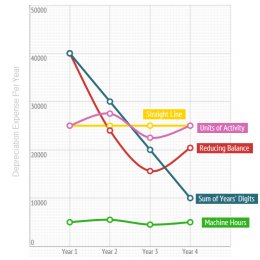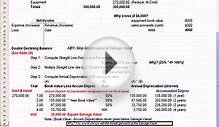# Financial Accounting depreciation methods

Cost of a fixed asset must be charged to the income statement in a manner that best reflects the pattern of economic use of assets.

Topic Contents:

## Types of depreciation

Common methods of depreciation are as follows:

## Impact of using different depreciation methods

The total amount of depreciation charged over an asset's entire useful life (i.e. depreciable amount) is the same irrespective of the choice of depreciation method. The adoption of a particular depreciation method does however effect the amount of depreciation expense charged in each year of an asset's life.

Following diagram illustrates the effect of using different depreciation methods on yearly depreciation expense:

The above illustration is based on the following information:

Cost of fixed assetalign="center"x"> \$100, 000 Useful Life 4 Years Reducing Balance DepreciationDepreciation charge is reduced by 40% in each period (i.e. the rate used in this example) until the last year in which the entire un-depreciated amount is charged off. Units of Activity Depreciation Depreciation charge varies in line with the change in number of machine hours consumed each year.

## Comparison of Depreciation Methods

Advantages Disadvantages May not reflect the true pattern of asset's economic benefits. Appropriate where the usefulness of an asset declines over its useful life (e.g. IT equipment). The rate of depreciation selected is subject to bias Easier to understand

The effect of decrease in depreciation expense compared to reducing balance method.

More difficult to calculate. Most accurately reflects the pattern of consumption of economic benefits.

Suitable in case of fixed assets that depreciate in proportion to units of activity rather than just the passage of time.

Difficult to determine and measure a reasonable basis of activity
##### YOU MIGHT ALSO LIKEDepreciation MethodsDepreciationFinancial Accounting 11: Depreciation Methods

### Related posts

#### Financial Accounting definition PDF

DECEMBER 08, 2023

In financial accounting, a liability is defined as the future sacrifices of economic benefits that the entity is obliged…